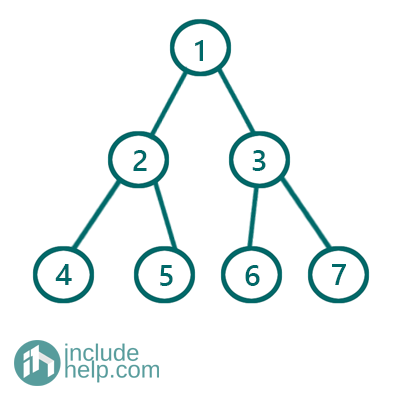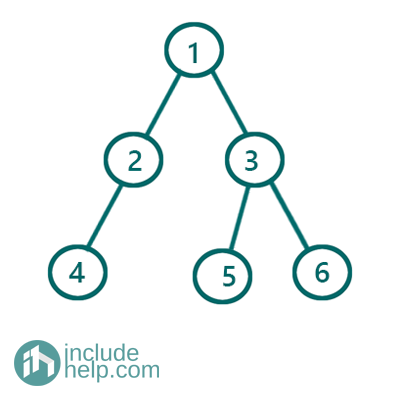# Perfect Binary Tree

In this article, we are going to see what Perfect Binary Tree is and how to check whether a binary tree is perfect or not?
Submitted by Radib Kar, on August 03, 2020

Prerequisite: Binary Tree

## What is a Perfect Binary Tree?

A Perfect Binary Tree is a special kind of binary tree where each and every internal node has exactly 2 children and all the leaf nodes lie at the same level.

For example, the below binary tree is a perfect binary tree whereas the second one is not.

Example 1:Figure 1: Perfect Binary Tree

The above example is a Perfect Binary Tree as every internal node in this has exactly two children and all the leaf nodes lie at the same level.

Example 2:Figure 2: Binary Tree which is Not a Perfect Binary Tree

The above binary tree is not a perfect binary tree as all its internal nodes don't have exactly 2 children though all leaf nodes lie in the same level. The node having value 4 has only one child.

## Properties for Perfect binary tree

There are few properties for Perfect binary tree which have been listed below:

Say,

Number of Total Nodes = N

Number of internal Nodes= I

Number of leaf Nodes= L

Height of the tree is: h

1. Number of total nodes in the perfect tree, N = 1+2+22+…+2h =2h + 1 – 1.
2. Number of leaf nodes in the perfect tree, L= 2h
3. Height of the perfect binary tree is of range Θ(log2(n)).

## Check whether a Binary Tree is Perfect binary tree or not

To check whether a binary tree is perfect or not can be done by using perfect tree properties. As we saw that we can find a number of nodes if we know the height of the binary tree. So we simply count the nodes using recursion and check whether it follows the rule.

```#include <bits/stdc++.h>
using namespace std;

class TreeNode {
public:
int val;
TreeNode* left;
TreeNode* right;

TreeNode(int v)
{
val = v;
left = NULL;
right = NULL;
}
};

int height(TreeNode* root)
{
if (!root)
return 0;

return 1 + max(height(root->left), height(root->right));
}

int count_no_of_nodes(TreeNode* root)
{
if (!root)
return 0;

return 1 + count_no_of_nodes(root->left) + count_no_of_nodes(root->right);
}

//Perfect Binary Tree checking
bool is_Perfect_Binary_Tree(TreeNode* root)
{

int h = height(root) - 1;
int total_no_of_nodes = count_no_of_nodes(root);
return pow(2, h + 1) - 1 == total_no_of_nodes;
}

int main()
{
//Example 1 Tree is built
TreeNode* root1 = new TreeNode(1);

root1->left = new TreeNode(2);
root1->right = new TreeNode(3);
root1->left->left = new TreeNode(4);
root1->left->right = new TreeNode(5);
root1->right->left = new TreeNode(6);
root1->right->right = new TreeNode(7);

if (is_Perfect_Binary_Tree(root1))
cout << "The tree is Example 1 is a Perfect Binary Tree\n";
else
cout << "The tree is Example 1 is not a Prefect Binary Tree\n";

//Example 2 Tree is built
TreeNode* root2 = new TreeNode(1);
root2->left = new TreeNode(2);
root2->right = new TreeNode(3);
root2->left->left = new TreeNode(4);
root2->right->left = new TreeNode(5);
root2->right->right = new TreeNode(6);

if (is_Perfect_Binary_Tree(root2))
cout << "The tree in Example 2 is a Perfect Binary Tree\n";
else
cout << "The tree in Example 2 is not a Perfect Binary Tree\n";

return 0;
}
```

Output:

```The tree is Example 1 is a Perfect Binary Tree
The tree in Example 2 is not a Perfect Binary Tree
```

What's New (MCQs)

Top Interview Coding Problems/Challenges!

Languages: » C » C++ » C++ STL » Java » Data Structure » C#.Net » Android » Kotlin » SQL
Web Technologies: » PHP » Python » JavaScript » CSS » Ajax » Node.js » Web programming/HTML
Solved programs: » C » C++ » DS » Java » C#
Aptitude que. & ans.: » C » C++ » Java » DBMS
Interview que. & ans.: » C » Embedded C » Java » SEO » HR
CS Subjects: » CS Basics » O.S. » Networks » DBMS » Embedded Systems » Cloud Computing
» Machine learning » CS Organizations » Linux » DOS
More: » Articles » Puzzles » News/Updates

© https://www.includehelp.com some rights reserved.